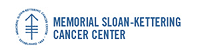## Memorial Sloan-Kettering Cancer Center, Dept. of Epidemiology & Biostatistics Working Paper Series

#### Title

The Bayesian two-sample t-test

#### Abstract

In this article we show how the pooled-variance two-sample t-statistic arises from a Bayesian formulation of the two-sided point null testing problem, with emphasis on teaching. We identify a reasonable and useful prior giving a closed-form Bayes factor that can be written in terms of the distribution of the two-sample t-statistic under the null and alternative hypotheses respectively. This provides a Bayesian motivation for the two-sample t-statistic, which has heretofore been buried as a special case of more complex linear models, or given only roughly via analytic or Monte Carlo approximations. The resulting formulation of the Bayesian test is easy to apply in practice, and also easy to teach in an introductory course that emphasizes Bayesian methods. The priors are easy to use and simple to elicit, and the posterior probabilities are easily computed using available software, in some cases using spreadsheets.

#### Disciplines

Statistical Methodology | Statistical Theory

COinS